# MCQs on Electrical Machines

##### Page 40 of 114. Go to page 1 2 3 4 5 6 7 8 9 10 11 12 13 14 15 16 17 18 19 20 21 22 23 24 25 26 27 28 29 30 31 32 33 34 35 36 37 38 39 40 41 42 43 44 45 46 47 48 49 50 51 52 53 54 55 56 57 58 59 60 61 62 63 64 65 66 67 68 69 70 71 72 73 74 75 76 77 78 79 80 81 82 83 84 85 86 87 88 89 90 91 92 93 94 95 96 97 98 99 100 101 102 103 104 105 106 107 108 109 110 111 112 113 114
01․ In a transformer zero voltage regulation is achieved at a load power factor which is
zero
unity
lagging

At leading power factor the voltage regulation can be negative or zero. This can be found from this equation % regulation = εxcosθ - εrsinθ

02․ A transformer has resistance and reactance in per unit as 0.01 and 0.04 respectively. Its voltage regulation for 0.8 power factor lagging and leading will be
6% and -4%
3.2% and -1.6%
4.8% and -3.2%
none of the above

Voltage regulation for lagging power factor = (R cosθ + X sinθ)×100 Voltage regulation for 0.8 lagging power factor = (0.01×0.8+0.04×0.6)×100 = 3.2% Voltage regulation for leading power factor = (R cosθ - X sinθ)×100 Voltage regulation for 0.8 leading power factor = (0.01×0.8 - 0.04×0.6)×100 = -1.6%

03․ The constant losses in transformer is/are
copper loss.
eddy current loss.
hysteresis loss.
both 2 and 3.

Eddy current loss and hysteresis loss are almost independent of load, significantly depending on supply voltage and frequency. As the flux density or flux is constant for a given voltage and frequency, eddy current loss and hysteresis loss remain constant at any load. Therefore, these losses are called constant losses. Copper loss varies as the square of load current and called variable loss.

04․ In a transformer, hysteresis and eddy current losses depend upon
maximum flux density
supply frequency
both 2 and 3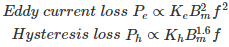Therefore, in a transformer, hysteresis and eddy current losses depend upon both maximum flux density and supply frequency.

05․ In a transformer operating at constant voltage if the input frequency increases, the core loss
increase.
decrease.
remains constant.
none of the above.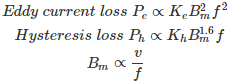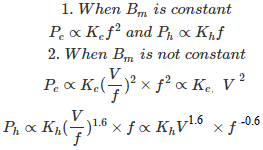06․ If the frequency of input voltage of a transformer is increased keeping the magnitude of voltage unchanged, then
both hysteresis loss(Ph) and eddy current loss(Pe) will increase
Ph will increase but Pe will decrease
Pe will increase but Ph will decrease
Ph will decrease but Pe will remain same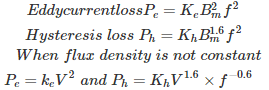Therefore, hysteresis loss will decrease but eddy current loss will remain same.

07․ 1 KVA, 230 V, 50 Hz, single phase transformer has an eddy current loss of 30 watts. The eddy current loss when the transformer is excited by a dc source of same voltage will be
30 W
more than 30 W
less than 30 W
zero watt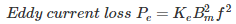Eddy current loss is directly proportional to supply frequency. Therefore, for dc source, frequency is zero and eddy current loss is also zero.

08․ A transformer has hysteresis loss of 30 W, at 240 V, 60 Hz. The hysteresis loss at 200 V, 50 Hz will be
28 W.
25 W.
30 W.
36 W.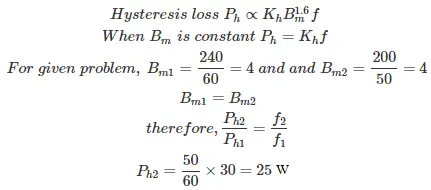09․ A single phase transformer when supplied from 220 V, 50 Hz has eddy current loss of 50 W. If the transformer is connected voltage of 330 V, 75 Hz, the eddy current loss will be
50 W
75 W
112.5 W
168.75 W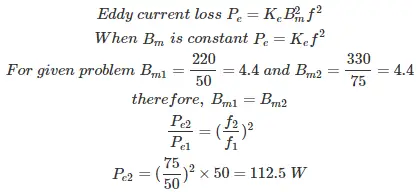10․ The full load copper loss and iron loss of transformer are 6400W and 5000W respectively. The copper loss and iron loss at half load will be respectively?
3200 W and 2500 W
3200 W and 5200 W
1600 W and 1250 W
1600 W and 5000 W

Iron losses do not depend on the load, iron losses remain constant for any load. Therefore iron losses are considered as constant losses. Copper losses vary as square of load current and these are considered as variable losses.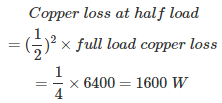<<<3839404142>>>